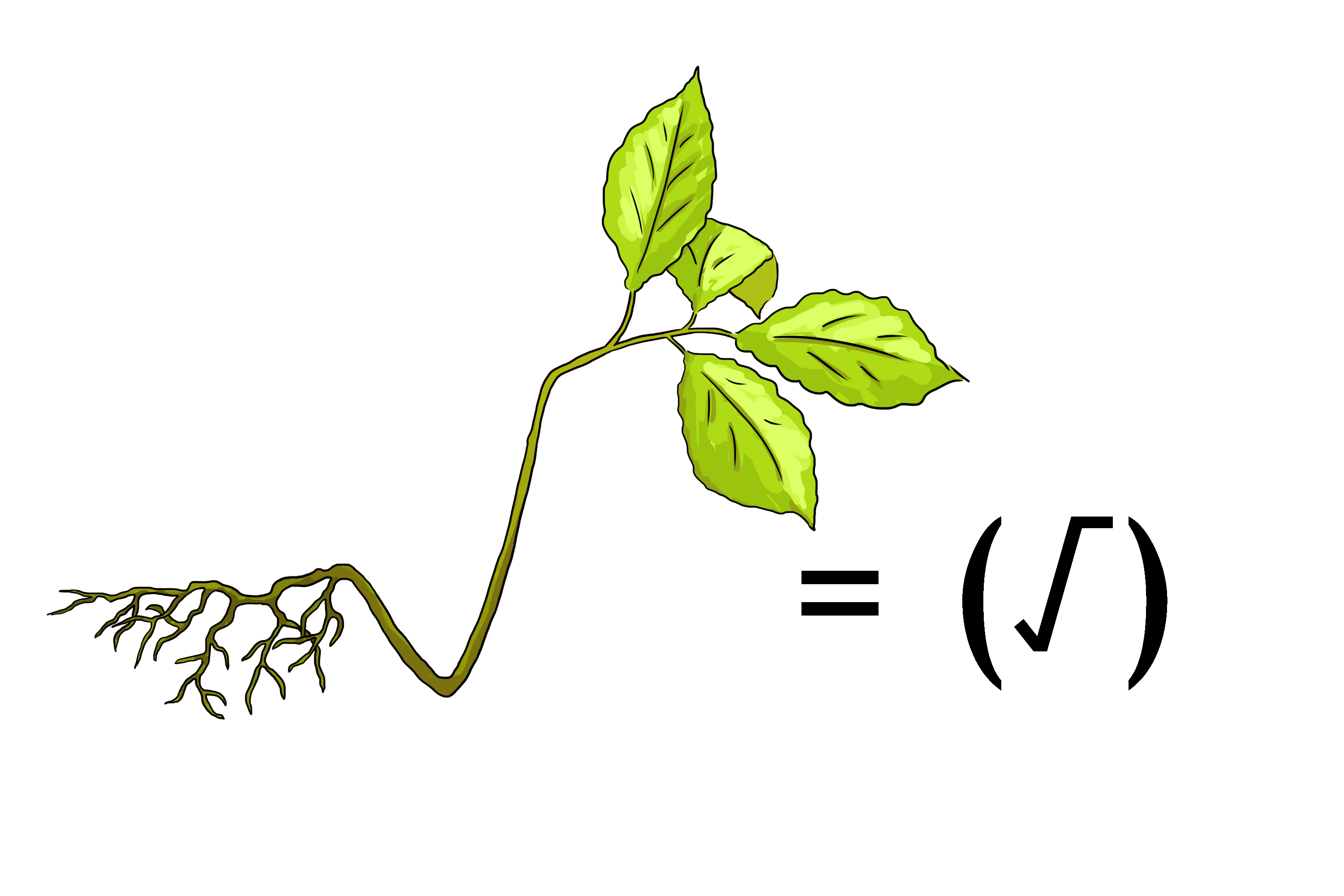# Roots

## Roots =sqrt(\  )

This symbol   sqrt(\  )=  RootThis tiny plant needs lots of roots to grow.

But what does sqrt really mean?

Use simple numbers that you know to work it out:

9\^(1/2)=\root2\9

So sqrt means

What do we have to multiply by itself 2 times to get 9

And in this case its 3  i.e. 3xx3=9

9\^(1/2)=\root2\9=3